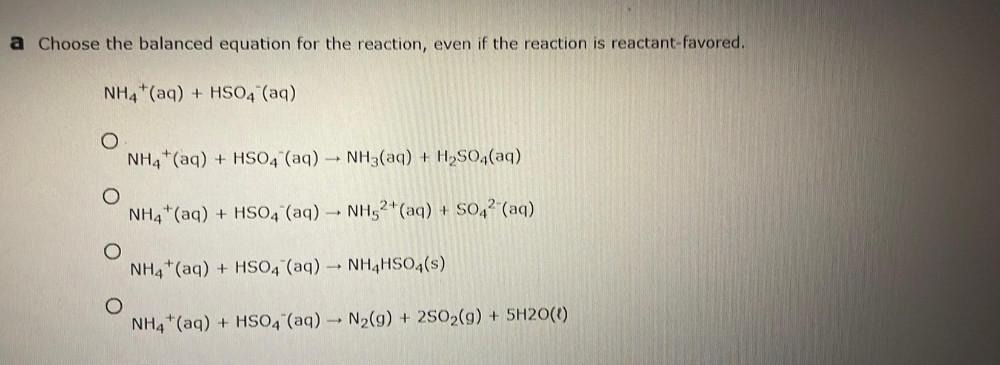Question:

# Choose the balanced equation for the reaction, even if the

Last updated: 7/25/2022Choose the balanced equation for the reaction, even if the reaction is reactant-favored. NH₄⁺(aq) + HSO₄‾(aq) A. NH₄⁺(aq) + HSO₄‾(aq) –> NH(aq) + H₂SO₄(aq) B. NH₄⁺(aq) + HSO₄‾(aq) -> NH₅²⁺(aq) + SO₄²‾(aq) C. NH₄⁺(aq) + HSO₄‾(aq) -> NH₄HSO₄(s) D. NH₄⁺(aq) + HSO₄‾(aq) -> N₂(g) + 2SO₂(g) + 5H₂O(l)